# How to change or convert text to number in Excel?

Sometimes the values in your Excel worksheets appear to be numbers, but they do not add up, do not multiply, and cause mistakes in the formulae that you are using. This occurs frequently because numbers are formatted as text in the document.

In many situations, Microsoft Excel is intelligent enough to automatically translate numerical sequences that have been imported from other programs into actual numbers. However, occasionally the numbers are left formatted as text, which might cause a variety of problems in your spreadsheets.

While the numbers are kept as text, you won't be able to do jobs in Excel like mathematical computations, construct charts from the values, or organize them into ranges. You also won't be able to use these features.

In this tutorial, we are going to show different methods to convert text to number.

## Using the "Convert to Number" Option

When an apostrophe is placed before a number, the number is converted to text. A green triangle will appear in the upper left corner of the cell in these instances.

### Step 1

In our example, we have some numbers formatted as text in Excel, as shown in the following screenshot.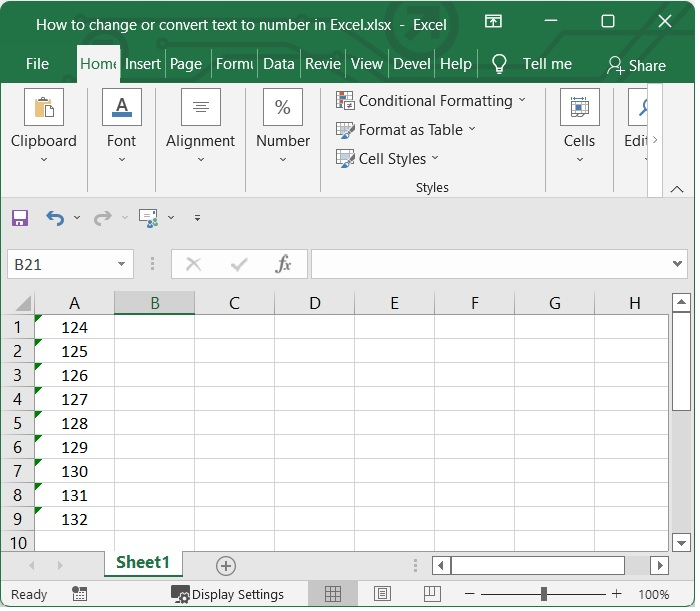### Step 2

Select all of the cells whose values you want to convert from text to numbers, and then click the Convert button.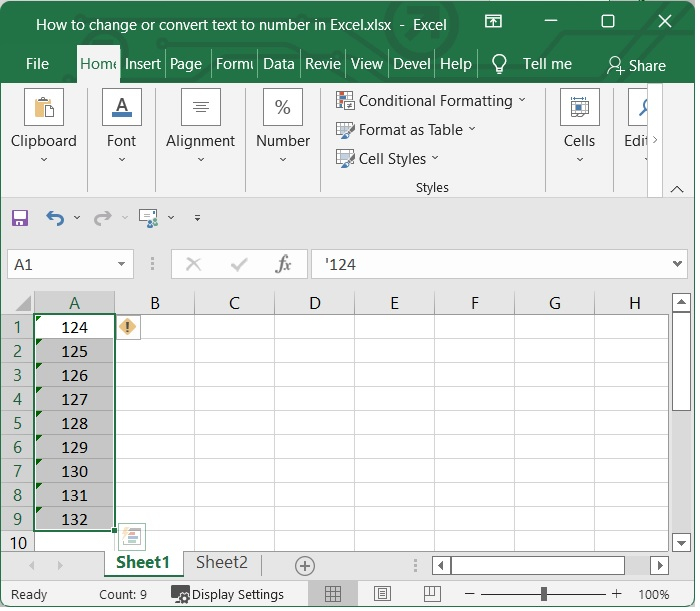### Step 3

Click on the diamond-shaped yellow icon in the top right corner. From the list that comes up, choose Convert to Number. See the below given image.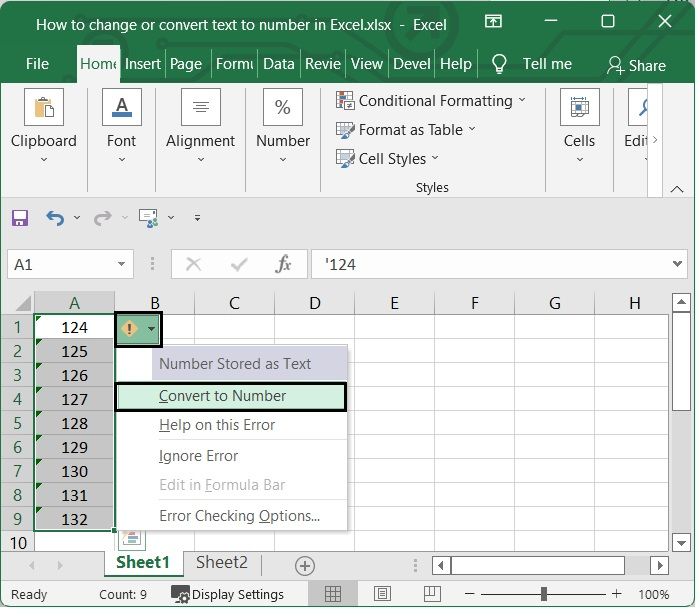It will be converted to number format.

## Using the "Paste Special" Option

Let's see how you can use the "Paste Special" option to convert text to number in Excel.

### Step 1

Put a "1" in any cell on the worksheet that is empty. Ensure that it is presented in the form of a number. See the following screenshot.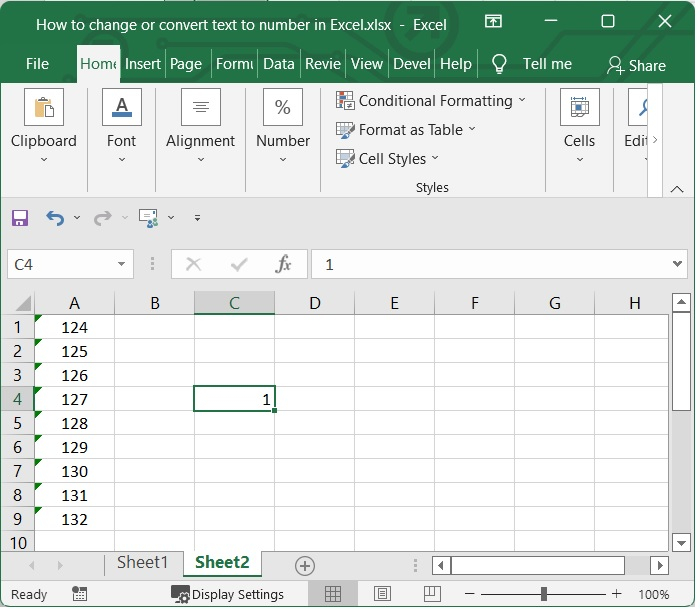### Step 2

Copy the cell that contains number 1. Choose the cells whose contents you wish to change from text to numbers. Then right click and then choose Paste Special from the list.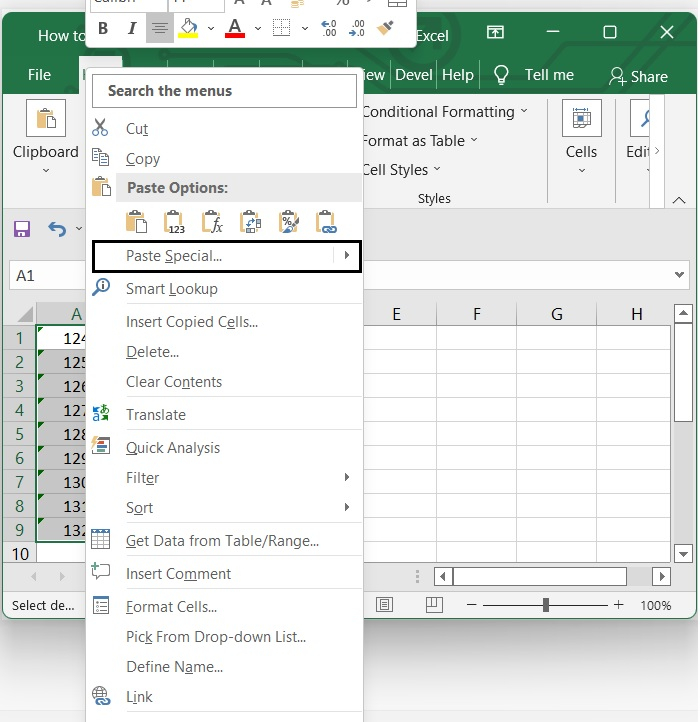### Step 3

Within the Paste Special dialogue box, navigate to the Operation category and make your selection there. Then click on OK. See the below given image.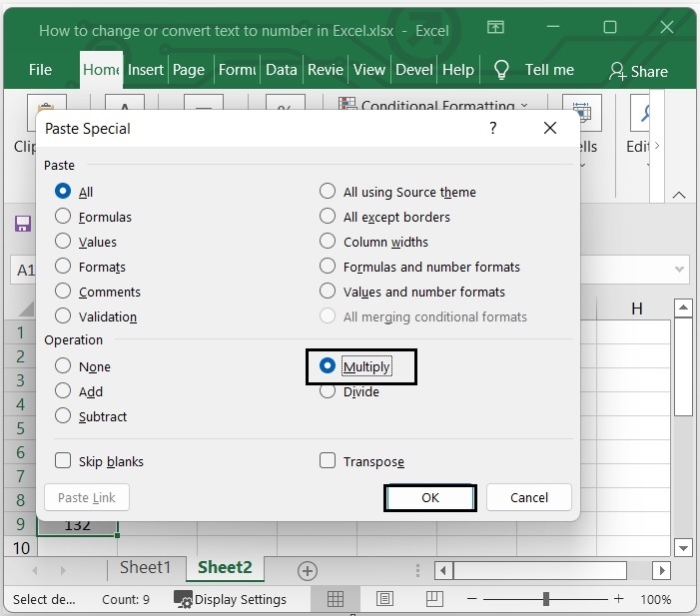After click on OK, the text will be converted to number. See the below given image.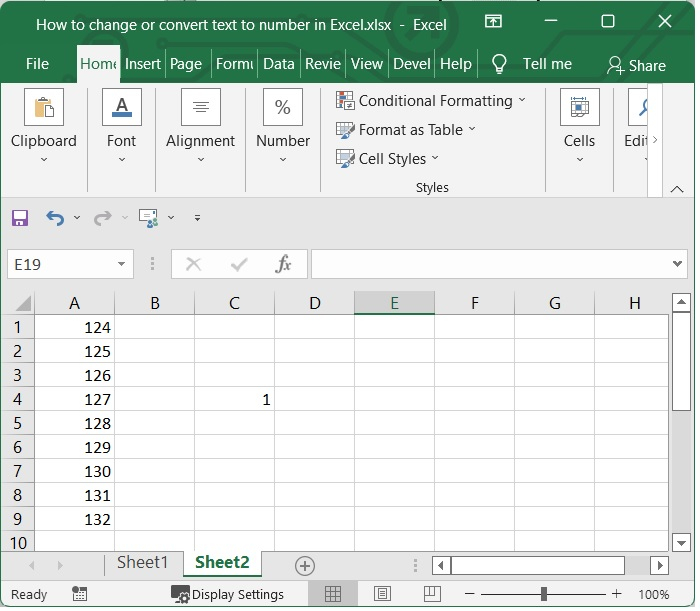## Using the VALUE Function

You can use the VALUE function to convert straightforward text values to corresponding numeric values.

### Step 1

Considering the previous example, select the number in the cell and add the below given formula. Then press enter.

=VALUE(A1)


See the below given image.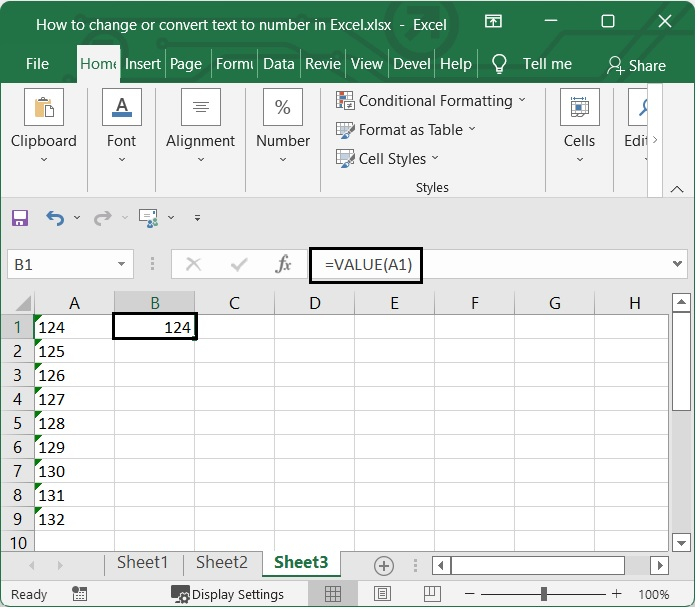### Step 2

drag the fill handle over the cells that you want to apply this formula to. Take a look at the following image.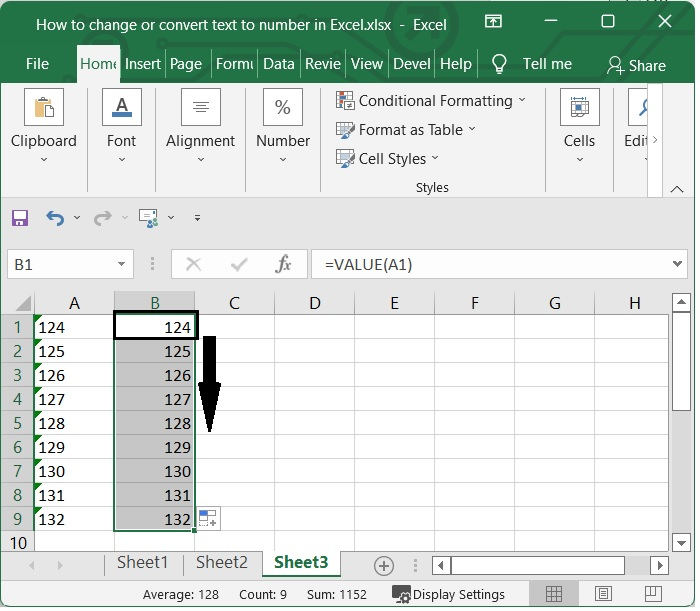## Conclusion

In this tutorial, we explained different methods that you can use in Excel to convert text to numbers.

Updated on: 06-Feb-2023

261 Views